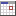# Savings annuity calculator with graph

This tool calculates the savings annuity also known as ordinary annuity, where regular deposits are made at the end of the payment period. The savings annuity calculates the future value of a stream of equal payments made at regular intervals over a specified period of time at a given interest rate.

It can be calculated using the following equation:

 FV = PMT * ((1 + R / N)N * T - 1) or FV = PMT * ((1 + r)N * T - 1) R / N rFV = N * T * PMT + I = A + I
r = R / N

where:
FV = Future value
I = Interest amount
A = Total payment amount
R = Nominal interest rate per year (as a decimal, not in percentage)
r = Interest rate per period (as a decimal, not in percentage)
T = Time period in years
N = Number of compounding periods in one year
PMT = Periodic payment amount, paid at the end of each payment period

Example:
John has an savings annuity with a term of 25 years, payment period is monthly with a payment size of \$50.
The nominal annual interest is 6%.

Question:
Find the future value (FV) and the earned interest (I)?
 FV = 50 * ((1 + 0.06 / 12)12 * 25 - 1) 0.06 / 12Future value FV = \$34649.70
Interest amount I = FV - N * T * PMT = 34649.70 - 12 * 25 * 50 = \$19649.70

This tools also calculates the payment amount (PMT), interest (I) and future value (FV) for each componding period and plot these data into a single graph, for example:
```==============================================================================
Period     Payment amount (PMT)     Interest earned (I)     Future value (FV)
==============================================================================
1                    50.00                    0.00                 50.00
2                   100.00                    0.25                100.25
3                   150.00                    0.75                150.75
4                   200.00                    1.51                201.51
5                   250.00                    2.51                252.51
:                       :                       :                     :
295                 14750.00                18800.01              33550.01
296                 14800.00                18967.76              33767.76
297                 14850.00                19136.59              33986.59
298                 14900.00                19306.53              34206.53
299                 14950.00                19477.56              34427.56
300                 15000.00                19649.70              34649.70
```
 Previous slide Next slide

This savings annuity calculator has many features:• Calculate the future value (FV) using equation:
 FV = PMT * ((1 + R/N)N * T - 1) R / N• Calculate the periodic payment amount (PMT) using equation:
 PMT = FV * (R/N) and FV = N * T * PMT + I (1 + (R / N))N * T -1Note:
A sinking fund is when we know the future value of the annuity and wish to calculate the periodic payment amount.

• Calculate the nominal interest rate (R) per year (as a decimal, not in percentage) using equation:
 FV = (1 + (R / N))N * T - 1 PMT R / NNote:
Keep substituting different values for R until we get successively closer to the desired value of FV / PMT.

• Calculate the time period (T) in years using equation:
 T = log((R / N) * ((FV / PMT) + (N / R))) and FV = N * T * PMT + I N * log(1 + (R / N))• Calculate the compounding periods (N) in one year using equation:
 N = log(((FV * r) / PMT) + 1) and FV = N * T * PMT + I T * log(1 + r)Note:
r = interest rate per period

For example, if you borrow \$1000 for 2 years at 12% interest compounded quarterly, you must divide the annual interest rate by 4 to obtain the interest rate per period
(r = R / N = 12 / 4 = 3%).

• Calculate the effective interest rate (Re) per year (as a decimal, not in percentage) using equation:
Re = (1 + (R / N))N   - 1          or          Re = (1 + r)N   - 1

Note:
The effective interest rate is the equivalent rate of compound interest earned over a period of one year for a nominal interest rate per year which is compounded twice or more over the year.

• Let you specify the compounding period:
• daily
360, 364, 365 or 366 times per year
• weekly
52 times per year
• bi-weekly (every two weeks)
26 times per year
• semi-monthly (twice a month)
24 times per year
• monthly
12 times per year
• bi-monthly (every two months)
6 times per year
• quarterly
4 times per year
• semi-annually (twice a year)
2 times per year
• yearly
1 time per year

• Let you specify the time period in two ways:
• Method 1: Enter the time period by number of: days, weeks, bi-weeklies, semi-monthlies, months, bi-monthlies, quarters, semi-annuallies or years.
• Method 2: Enter a from date and to date. Both dates can also be included or excluded.

• Set the number of days in a year to 360, 364, 365 or 366 days.

• Draw two graphs in a single plot (see images above).
• Graph 1: Future value (FV) - Month
• Graph 2: Future value (FV) / Interest earned (I) - Month

To show/hide a graph click the name in the plot legend.
Press button "Make plot image" to create an image which can be saved.

## Input savings annuity calculator with graph:

 Calculate:Future value (FV)Periodic payment amount (PMT)Nominal annual interest rate (R)Time period (T)Compounding period (N) Future Value (FV)*:Periodic payment amount (PMT): *:Nominal annual interest rate (R) *: %   per year Compounding period (N):dailyweeklybi-weekly (every two weeks)semi-monthly (twice a month)monthlybi-monthly (every two months)quarterlysemi-annually (twice a year)yearly Time period (T) *:daysweeksbi-weeklies (every two weeks)semi-monthlies (twice a month)monthsbi-monthlies (every two months)quarterssemi-annuallies (twice a year)years From / To dateFrom date:To date:Include From / To dateInclude from date: Include to date: Days in year:360364365366 Chart:Stack bar chartLine chart * = required